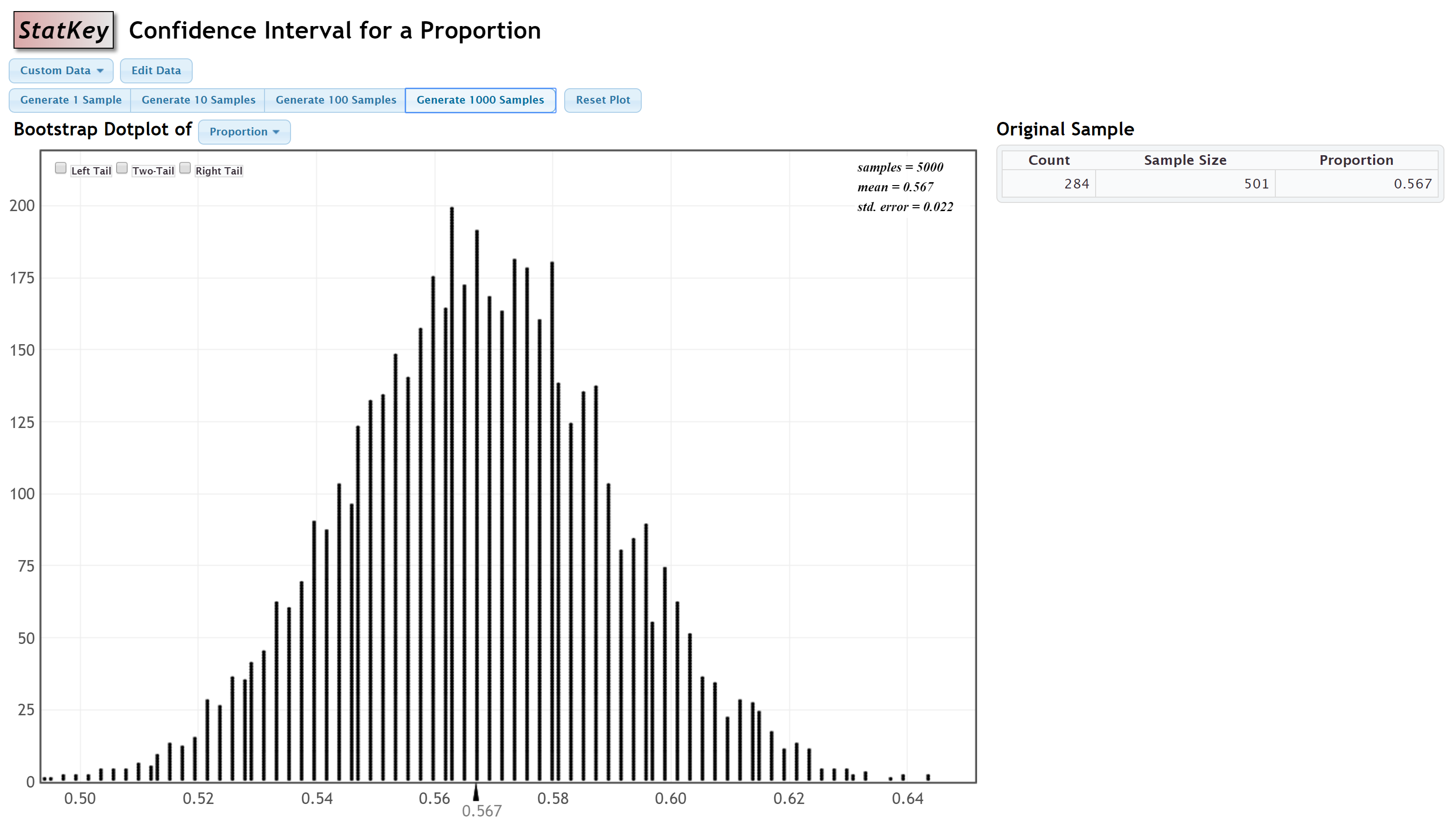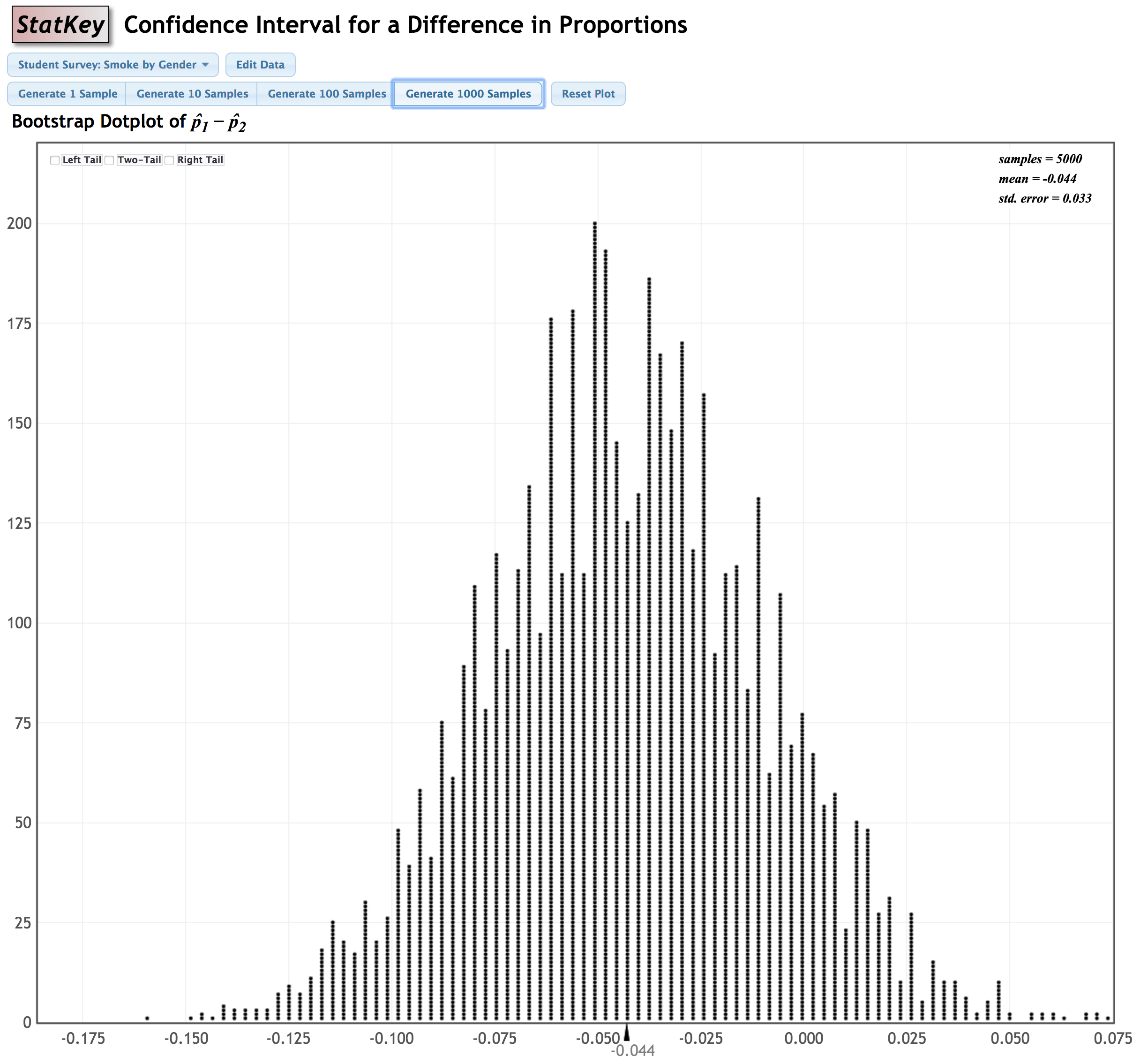# 7.4.2 - Confidence Intervals

7.4.2 - Confidence Intervals

## Standard Normal Distribution Method

The normal distribution can also be used to construct confidence intervals. You used this method when you first learned to construct confidence intervals using the standard error method. Recall the formula you used:

95% Confidence Interval
$sample\;statistic \pm 2 (standard\;error)$

The 2 in this formula comes from the normal distribution. According to the 95% Rule, approximately 95% of a normal distribution falls within 2 standard deviations of the mean.

Using the normal distribution, we can conduct a confidence interval for any level using the following general formula:

General Form of a Confidence Interval
sample statistic $\pm$ $z^*$ (standard error)
$z^*$ is the multiplier

The $z^*$ multiplier can be found by constructing a z distribution in Minitab Express.

## z* Multiplier for a 90% Confidence Interval

What z* multiplier should be used to construct a 90% confidence interval?

For a 90% confidence interval, we would find the z scores that separate the middle 90% of the z distribution from the outer 10% of the z distribution:

For a 90% confidence interval, the $z^*$ multiplier will be 1.64485.

# 7.4.2.1 - Video Example: 98% CI for Mean Atlanta Commute Time

7.4.2.1 - Video Example: 98% CI for Mean Atlanta Commute Time

Construct a 98% confidence interval to estimate the mean commute time in the population of all Atlanta residents.

This example uses a dataset is built in to StatKey: Confidence Interval for a Mean, Median, Std. The dataset is titled 'Atlanta Commute.'

# 7.4.2.2 - Video Example: 90% CI for the Correlation between Height and Weight

7.4.2.2 - Video Example: 90% CI for the Correlation between Height and Weight

Construct a 90% confidence interval to estimate the correlation between height and weight in the population of all adult men.

# 7.4.2.3 - Example: 99% CI for Proportion of Students Female

7.4.2.3 - Example: 99% CI for Proportion of Students Female

Scenario: Data were collected from a representative sample of 501 World Campus STAT 200 students. In that sample, 284 students were female and 217 were male. Construct a 99% confidence interval to estimate the proportion of all World Campus students who are female.

StatKey was used to construct a sampling distribution using bootstrapping methods:Because this distribution is approximately normal, we can approximate the sampling distribution using the z distribution. We will use the standard error, 0.022, from this distribution.

The original sample statistic was $\widehat p =\frac{284}{501}=0.567$.

We can find the $z^*$ multiplier by constructing a z distribution to find the values that separate the middle 99% from the outer 1%:The $z^*$ multiplier is 2.57583

Recall the general form of a confidence interval: sample statistic $\pm$ $z^*$ (standard error) where $z^*$ is the multiplier. So in this case we have...

$0.567 \pm 2.57583 (0.022)$

$0.567 \pm 0.057$

$[0.510, 0.624]$

I am 99% confident that the proportion of all World Campus students who are female is between 0.510 and 0.624

# 7.4.2.4 - Example: 95% CI for Difference in Proportion of Smokers by Sex

7.4.2.4 - Example: 95% CI for Difference in Proportion of Smokers by Sex

Construct a 95% confidence interval to estimate the difference between the proportion of all females who smoke and the proportion of all males who smoke.

This dataset is built in to StatKey: Confidence Interval for Difference in Proportions. It is the Student Survey: Smoke by Gender dataset.

#### Original Sample

Group Count Sample Size Proportion
Female 16 169 0.095
Male 27 193 0.140
Female-Male -11 n/a -0.045

StatKey was used to construct a bootstrap sampling distribution:Because this distribution is approximately normal, we can approximate the sampling distribution using the z distribution. We will use the standard error, 0.033, from this distribution.

The original sample statistic was $\widehat p_f - \widehat p_m = \frac{16}{169} - \frac{27}{193} = -0.045$

We can find the $z^*$ multiplier for a 95% confidence interval using Minitab Express. This will be the values on a z distribution that separate the middle 95% from the outer 5%. (Note: You could apply the Empirical Rule and use a multiplier of 2, but the value found using Minitab Express will be more precise)The $z^*$ multiplier is 1.95996.

Recall the general form of a confidence interval: sample statistic $\pm$ $z^*$ (standard error) where $z^*$ is the multiplier. So in this case we have...

$-0.045 \pm 1.95996(0.033)$

$-0.045 \pm 0.065$

$[-0.110,0.020]$

I am 95% confident that the difference in the population between the proportion of females who smoke and the proportion of males who smoke (i.e., $p_f-p_m$) is between -0.110 and 0.020.

  Link ↥ Has Tooltip/Popover Toggleable Visibility